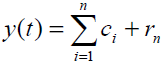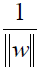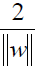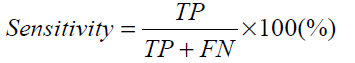## Biomedical Research

All submissions of the EM system will be redirected to Online Manuscript Submission System. Authors are requested to submit articles directly to Online Manuscript Submission System of respective journal.

- Biomedical Research (2013) Volume 24, Issue 3

## Noninvasive diagnosis of atherosclerosis by using empirical mode decomposition, singular spectral analysis, and support vector machines.

Department of Computer Engineering, Fatih University, 34500, Buyukcekmece, Istanbul, Turkey

*Corresponding Author:
Department of Computer Engineering
Fatih University
Istanbul, Turkey

Accepted date: March 25 2013

Citation: Tufan K. Noninvasive diagnosis of atherosclerosis by using empirical mode decomposition, singular spectral analysis, and support vector machines. Biomedical Research 2013; 24 (3): 303-312.

Visit for more related articles at Biomedical Research

### Abstract

In recent years, the use of Doppler ultrasound sonography for the diagnosis of atherosclerosis has increased. However, parameters provided by the commercially available Doppler ultrasound units are inadequate to compete with the gold standard method, catheter angiography. Therefore, developing a new method based on Doppler ultrasound sonography remains a hot topic among researchers. In this study, a diagnostic method based on empirical mode decomposition, singular spectral analysis, and support vector machines is proposed. The nonlinear and non-stationary nature of the Doppler ultrasound sonogram is utilized in the analysis. Empirical mode decomposition is a suitable tool for such signals. Singular spectral analysis is used to construct the classifications feature set. Finally, support vector machine classification is applied. When compared with clinical findings and similar methods, the proposed method provides excellent results.

## Keywords

Doppler ultrasound, atherosclerosis, empirical mode decomposition, nonlinear analysis, pattern recognition, singular spectrum analysis, support vector machines.

## Introduction

Loss of elasticity of the arteries, or atherosclerosis, is a common sickness wherein the wall of the artery thickens because of accumulated fatty materials such as fat, cholesterol, and calcium. When these materials accumulate on the inner surface of the artery, the artery starts to lose its elasticity, i.e., it cannot expand well. This condition affects both large and medium-sized arteries, such as carotid, coronary, and renal arteries.

There are different methods for diagnosing atherosclerosis. Among them, the catheter angiography, an invasive method, is accepted as the gold standard approach . However, besides being invasive, this approach also has other disadvantages, such as being relatively expensive, as well as non-versatile, due to the need for special equipment and expert personnel. Doppler sonography, which has been suggested as an alternative, has been used for decades to diagnose narrowing of the arteries. Nevertheless, direct application of this method has some inherent problems. More expressly, the parameters provided by the Doppler ultrasound sonogram sometimes become inadequate to diagnose plaque formation, especially during the preclinical phase. Therefore, further analysis of Doppler sonogram signals is required to increase the correct detection rate .

Studies conducted based on the sonogram recording acquired from the carotid artery are summarized herein. Spectral analysis of the sonogram reveals important features that cannot be obtained from the raw form of the signal. One of the early research studies based on signal analysis was conducted by Dirgenali, Kara, Erdogan, Okandan , who utilized the fast Fourier transform and autoregressive processing of the Doppler sonogram to classify healthy individuals and patients with atherosclerosis. The researchers obtained parameters from the spectral analysis of the sonogram and analyzed their statistical reliability. In subsequent studies, some pattern recognition tools were utilized in the classification of atherosclerosis. For feature extraction, spectral characteristics [5,6], wavelet analysis [6-8], nonlinear characteristics , and eigenvector methods  were used. For classification, different forms of artificial neural network [7,11], support vector machines [12,13], and hidden Markov models  were used. For feature size reduction, a principal component analysis method was applied in some studies .

The blood flow of arteries shows a nonlinear/nonstationary flow dynamic , and this chaotic nature of the flow makes it suitable for nonlinear analysis. Several studies related to the classification of atherosclerosis by using nonlinear analysis have been conducted. In these studies, the researchers extracted the largest Lyapunov exponent (LLE) and the correlation dimension (CD) as classification parameters . The results of these studies provided high classification accuracy with very low error rate. The results of these studies were encouraging enough to promote a search for alternative nonlinear methods.

In this study, the nonlinear characteristics of Doppler ultrasound sonograms obtained from the carotid arteries of healthy and sick subjects were used to obtain classification features. First, the complicated Doppler signal was decomposed into a finite number of intrinsic mode functions (IMF) by using empirical mode decomposition (EMD). This decomposition is effective because it is selfadaptive. Moreover, it is very convenient for analysis of non-stationary and nonlinear signals, as the breakdown process is performed by considering the local time-scale properties of the signal . The singular spectral analysis (SSA) method was utilized to produce the classification feature vector. SSA reveals the principal components of signal in time domain. In other words, the trend and oscillation components are revealed . The SSA method was used in this study as a feature extraction and reduction purpose. Instead of the entire IMF, only a few principal components obtained from each IMF were obtained and used as the feature vector; hence, a feature size reduction occurred.

Supervised learning is a machine learning approach where classification or regression rules are generalized from labeled training data. Support vector machines (SVM) is a supervised classification method that draws an optimal hyperplane between classes during the training phase . It is effectively applied for classification and regression purposes, and has been successfully applied in many biomedical classification problems in the literature [20- 22]. SVMs with different kernel functions were used in this study to demonstrate the most successful one for the classification of healthy and atherosclerosis Doppler sonograms.

This paper is organized into the following sections: In Chapter 2, the theoretical basements of EMD, SSA, and SVM are explained. In Chapter 3, the procedures applied in the study are described. Experimental results are given in Chapter 4. Chapter 5 provides the discussion and conclusion.

## Theory

Empirical Mode Decomposition

Empirical mode decomposition (EMD) is the name of the process that breaks down a time series signal into a finite number of scales, where each scale is called an intrinsic mode function (IMF) [17,23]. It still keeps the time domain, and does not rely on any modeling assumption. EMD is a very effective tool for analyzing time series, which are mostly non-stationary and/or nonlinear . In EMD, the signal x(t) is broken down into its IMFs. EMD has some important differences from almost all the methods used in data analysis that were discussed previously. Because it relies on the signal itself, EMD is adaptive. All sub-band decompositions are performed according to the signal. In the EMD approach, the signal y(t) is subdivided into IMFs, ci , such thatwhere rn is the residue of the signal y(t) ,which is decomposed into n IMFs.

The EMD filters out a signal into its IMFs, which fulfill the following criteria:

1. The number of local extremes and zero crossings may differ at most by one, or they are equal.

2. The mean values of the envelopes defined by the local minima and maxima must be zeros at any point.

Although the above conditions imply that an IMF represents a simply oscillatory mode that has constant amplitude and frequency, an IMF can have variable amplitude and frequency. The process that reveals the IMFs is called a shifting process. The basic steps of the shifting process are as follows:

1. Identify the local minima ad maxima of the signal given.

2. Join all local minima by cubic spline lines to form the lower envelope.

3. Connect all local maxima by cubic spline lines to form the upper envelope.

4. The mean value of the data surrounded by the upper and the lower envelopes is m1 . The first IMF (IMF1) is obtained by

h1=x(t)+m1 (2)

5. The h1 becomes the new data signal. The above steps are repeated to calculate the second IMF.

6. The shifting process is repeated until stoppage criterion is reached. If k time to decomposition occurs, then there are a total of k IMFs and one residue.

Traditionally, there are two different stoppage criteria [17, 23]. In this study, the stoppage criteria were determined by using the method described in . Successive filtering is stopped when the mean of the residue drops below a certain threshold value.

2.2. Singular Spectrum Analysis

Singular spectrum analysis (SSA), a powerful, nonparametric technique used in time series analysis and forecasting [26-28], is based on the singular value decomposition of a matrix constructed from time series. SSA is especially useful with time series that are noisy and of short duration . The assumption is that the time series is stationary, and the signal must be of short duration. SSA has many good applications, such as signal noise suppression, trend forecasting, quasi-periodic component detection, biomedical application econometrics, and engineering applications .

SSA is based on embedding a time series of x(t): t = 1,.., N (has N samples) into a vector space of M dimensions (M < < N ). The procedure is as follows:

1. Choose the embedded dimension size (M ).

2. Define L such that L = N -M +1

3. Construct the Hankel matrix A ( L ´ M ) by using mean correlated data y t:t = 1,2,...,N(3)

4. Determine the eigenvalues and eigenvectors from AT A. Eigenvalues are calculated and ordered in descending order λ12....λk For each eigenvalue, λi there is an eigenvector ,vi such that (AT A)viivi

5. Define a new series, , wi such that wi= Avi i=1,2,...M, . The length of each series is L . The new series are called latent variables, and the analysis is conducted over the latent variables.

Support Vector Machines

Support vector machines (SVM) is a supervised classifier that can successfully classify linear and nonlinear classification problems [19,32]. It can separate different labeled classes by describing a classification boundary, called a hyperplane in N-dimensional space. Because SVM is a supervised method, it has two steps—learning and testing phases. It is similar to the artificial neural network (ANN); both are kernel-based methods . By using the labeled training set, the SVM draws a hyperplane for classes. Then, the unused test set is given to the SVM for classification. SVM classifies the test set by using the previously obtained classification hyperplane. These hyperplanes are high dimensional spaces; the functional margin between classes is maximized to minimize the generalization error during the training time . The elements of classes nearest to the hyperplane are called support vectors .

Let us have a set x of n samples, where each sample has p features and a label c that indicates the class of the sample. For a two classes case, c = 1for class1 and c = -1for class2. The SVM defines the hyperplane that clearly identifies two regions, where each region has samples of one class while leaving a maximum gap between classes. A hyperplane that has an arbitrary size can be expressed as

w · x + b = 0 (4)

✓“ w ”: normalized weight vector that is perpendicular to the hyperplane

✓ “ · ” : the dot product operation

✓ “ x ” : a vector on the hyperplane

Let the hyperplane given in (4) be the hyperplane that optimally separates two classes. In addition, define the hyperplane that has elements of class1 nearest to the separation hyperplane given in (4). This hyperplane can formulated as

w · x + b = 1 (5)

Similarly, the hyperplane that has elements of class2 nearest to the desired classification hyperplane is w · x + b = - 1 (6)

The sample points that lie on the hyperplane described in (5) and (6) are called support vectors (Fig.1). Because the separation hyperplane is exactly in the middle of the support vectors, the distance between the separation hyperplane and the hyperplane given in (5) is.Similarly, the distance between the separation hyperplane and the hyperplane given in (6) is also.The total difference (distance between the nearest samples of two classes) between the support vectors of two classes isThe process of finding the support vectors and optimal separation hyperplane is an optimization problem. There is a single solution in quadratic optimization space for this problem.

Figure 1: Illustration of support vector machines for a two-class classification problem

Procedures

First, the data collection and the demographic information related to the data are summarized, and some important aspects of the data are explained. Then, offline procedures for classification of the healthy and atherosclerosis subjects are given in stepwise form.

The Doppler ultrasound sonograms of healthy subjects and subjects with atherosclerosis were analyzed and classified as shown in Fig. 2. The details of each step will be explained later. In summary:

1. Preprocessing: In this step, first, the line effects of the records are removed by using a 50 Hz notch filter. Then, each record is analyzed, one by one, to select two seconds of clear section.

2. Analysis and feature extraction: Selected sonogram sections are decomposed into sub-bands by using empirical mode decomposition. Then, singular spectral analysis is applied to obtain a feature vector.

3. Classification: Support vector machines are used to classify the obtained feature set.

4. Classification result: After classification, the results are compared with the clinical classification for appraising the success rate of the suggested method.

Collection of Data and Demographics

The Doppler ultrasound signals were recorded at Erciyes University Medical School Hospital Radiology Department, using a Toshiba PowerVision 6000 Doppler Ultrasound Unit equipped with a 10 MHz linear ultrasound probe. The insonation angle was kept constant at 45° on the longitudinal view. An I/O card was used to record the sonogram to a personal computer.

Data was acquired from the left carotid arteries of subjects. Subjects indicating early phase of atherosclerosis were further verified through angiography. The healthy subjects were chosen among young, nonsmoking volunteers with no signs of atherosclerosis. The demographics of the subjects are provided in Table 1; recording specifications are provided in Table 2. For further details regarding data recording and demographic information, please refer to .

After the data was recorded in a personal computer, the offline signal analysis and further classification processes were performed using MATLAB® (MATLAB7.6.0, R2008a, MathWorks Inc., Natick, MA, USA).

Preprocessing

In this step, there are two operations to prepare the Doppler ultrasound records for the feature extraction step. 1. Line effect removing: A 50 Hz notch filter is used to increase the signal-to-noise ratio of the Doppler ultrasound recordings.

Analysis and Feature Extraction

The number of successive divisions was found to be five during the EMD phase in this study. Therefore, including the residue, six sub-bands were obtained by dividing the original signal. Then, SSA was applied to each IMF and residue to calculate the eigenvalues. By using the Scree test method widely used in literature , the number of selected eigenvalues for each IMF and residue was determined to be five. Hence, a total of 30 eigenvalues were used to construct the feature vector. Finally, the feature set was constructed from the eigenvalues of 78 samples.

As an illustration, EMD decomposition results of one healthy subject and one atherosclerosis subject are provided in Fig. 3 and Fig. 4, respectively.

Implementation of classification

The SVM used in this study is briefly illustrated in Fig. 5. As the size of the constructed feature vector is 30, there are 30 nodes at the input part. The number of the support vector is set automatically during the training phase. In other words, the number of support vectors varies for each trial. In the given figure, the number of the support vector is 22. Because we have two classes, one output node is enough. Basic specifications of the SVM are provided in Table 3.

Experimental Results

In this study, a diagnostic classification method utilizing EMD, SSA, and SVM was developed to discriminate the Doppler ultrasound recordings of healthy and atherosclerosis subjects. Doppler ultrasound sonograms collected from 40 healthy volunteers and 38 subjects with atherosclerosis were classified by utilizing pattern recognition techniques that employ advanced signal processing tools for feature extraction.

A preprocessing step was conducted first, in which lownoise, 2-second sections of each recording were selected for analysis purposes. A notch filter was applied to remove the line effects. Then, EMD was used to divide the signals into sub-bands; EMD is effective in the analysis of data that is nonlinear and/or non-stationary. Continuous sub-band production was stopped after five iterations by applying the stopping criteria used in the literature. Thus, including the residue, a total of six sub-bands were obtained. SSA was performed for each sub-band, and eigenvalues were obtained. SSA decomposes a time series (here, each IMF and the residue of the sonogram) into the sum of a small number of independent components. In this study, SSA acted as a feature reduction operator, as it shrank the number of data points obtained at the EMD analysis. The eigenvalues were used to construct the classification set. As an example, the eigenvalues of one healthy and one atherosclerosis subject are shown in Fig. 6; these eigenvalues of the IMF1 and IMF2 sub-bands were plotted in logarithmic scale (base 10) for better visualization. Visual discrimination of these samples is possible, but for generalization, an expert system that reveals the hidden properties of these two classes is needed. Hence, SVM was utilized as a supervised classifier.

Figure 6: Illustration of SSA analysis results of one healthy subject and one sick subject

For SVM classification, linear kernel was chosen as the kernel function. The main reason is to start from the simple one; if it does not produce satisfactory results, then other kernel functions are tried. The linear kernel is simple because it is just a dot product operation, and it has fewer parameters than other functions. The user can set the scale factor and shift parameters, or they can be set automatically. In this study, they were set automatically.

A 10-fold analysis was performed to evaluate the performance of the classifier. Seventy samples were used for training purposes, and the rest were used for testing purposes. The results of this process are shown in Table 4; it can be seen that this classification was completely successful, with both the training and testing phases providing100% accuracy. The criteria for the performance evaluation are explained as follows.

The performance of the proposed system was measured with three parameters; accuracy, sensitivity, and specificity.

Test Accuracy(7)

Test Sensitivity(8)

Test Specificity(9)

where

TP (True Positive)

A subject who is proved clinically by the expert as having atherosclerosis is also classified as having atherosclerosis by the suggested method.

TN (True Negative)

A clinically diagnosed healthy subject is also classified as healthy by the proposed method.

FP (False Positive)

A clinically diagnosed healthy subject is classified as having atherosclerosis by the suggested diagnostic method.

FN (False Negative)

A subject who is proved clinically by the expert as having atherosclerosis is classified as healthy by the proposed method.

A second study was performed on the classification to determine the amount of data that would be enough to train the SVM classifier without decreasing the classification accuracy. When the number of training samples is decreased, the number of testing samples is automatically increased. A larger number of training samples means better generalization. The results of this analysis are shown in Table 5. First, the number of training samples chosen was 40, which is the 50% of the samples. This process was repeated 10 times; in each step, 40 samples were chosen randomly for the training set, and the remaining 38 samples were used to evaluate the testing accuracy. The accuracy of this test is the average of the 10 trials; as shown in Table 5, the accuracy of this setting was 99.21%. The number of training samples was then increased by 5% in every step, and the average accuracy for 10 trails was recorded. The test accuracy of the classifier was slightly less than 100% until the training samples comprised 70% of all samples. Then, while increasing the training sample size, 100% testing accuracy was achieved. These results show a consistency that up to 30% testing samples can be used.

## Discussion and conclusion

In recent years, Doppler ultrasound sonogram methods have been suggested for the diagnosis of the early phase of atherosclerosis, as an alternative to catheter angiography. Because direct application of the sonogram performs poorly, studies have been conducted using advanced signal processing and expert system classifiers.

In this study, the nonlinear and non-stationary structure of arterial blood flow was used for feature extraction. Because EMD is well suited to such signals, the classification performance was expected to be high. A Doppler ultrasound sonogram signal was decomposed into five IMFs and one residue, and then SSA was applied to calculate the eigenvalues. For each sub-band, five eigenvalues were calculated. Hence, a total of 30 eigenvalues were obtained that were used to construct the feature set. In order to classify the constructed feature set, SVM was utilized. SVM has been successfully applied in many biomedical classification problems in the literature. Linear kernel was chosen for its ease of application, and was used in the well-known 10-fold classification. In a 10-fold classification scheme, the data is divided into 10 groups, each having one-tenth of the total samples. In each fold, one group is used for testing and the remaining 90% are used for the testing phase. Among the 78 samples, eight were used for testing in each fold. Here, 100% average classification performance was achieved.

In this study, the limit for the training data that provides the best testing classification performance was also investigated. For this purpose, the amount of the training data starts from a low value (50% here), and increases gradually (5% here) in each step. The aim is to obtain the lowest training sample size (naturally the highest testing sample size) that gives 100% classification. This classification accuracy is achieved when the training sample size is 70% of the whole set. In other words, 30% of the samples are used for the testing. This is much higher than the 10-fold scheme.

When compared with other studies using the same data, the method suggested in this study has some advantages:

1. The proposed method is very simple and straightforward, which makes it suitable for application in similar problems. Moreover, it requires little time.

2. Because the linear kernel is used as the kernel function, the SVM classifier used in this study is easy to apply and needs few (it any) tuning operations.

3. It achieved 100% accuracy not only with the 10- fold method, which uses 90% of the samples for constructing the training dataset, but also with a lower training sample size. This result is an indicator of the efficiency of the suggested method for the discrimination of atherosclerosis subjects.

4. To the best of our knowledge, in this study, EMD and SSA were used successively for the first time in feature extraction of Doppler sonograms obtained from healthy and atherosclerosis subjects.

5. The short processing time makes it possible for the proposed system to be merged into real time systems used in clinics.

## Acknowledgement

The author thanks Dr. Sadık Kara for providing the research data.

## Conflict of Interest

The authors declare that they have no conflict of interest.